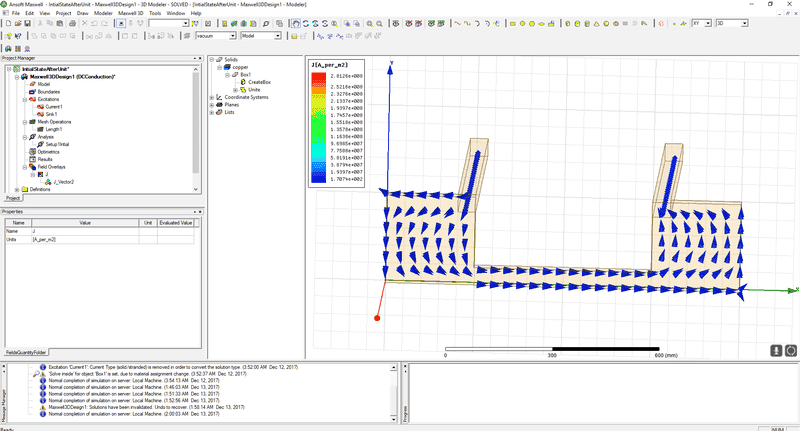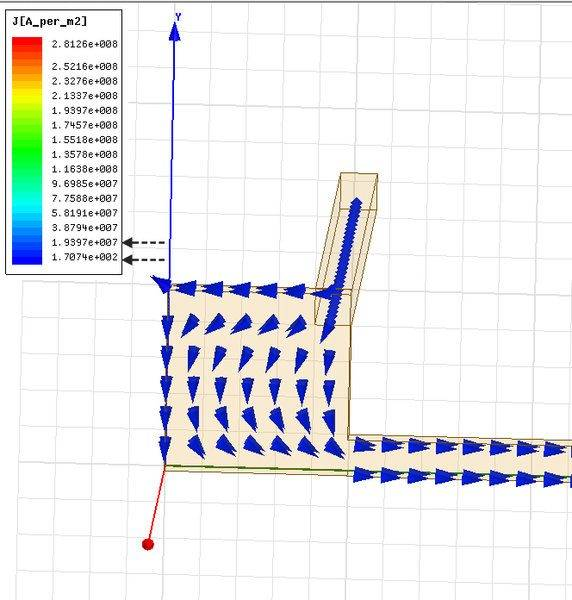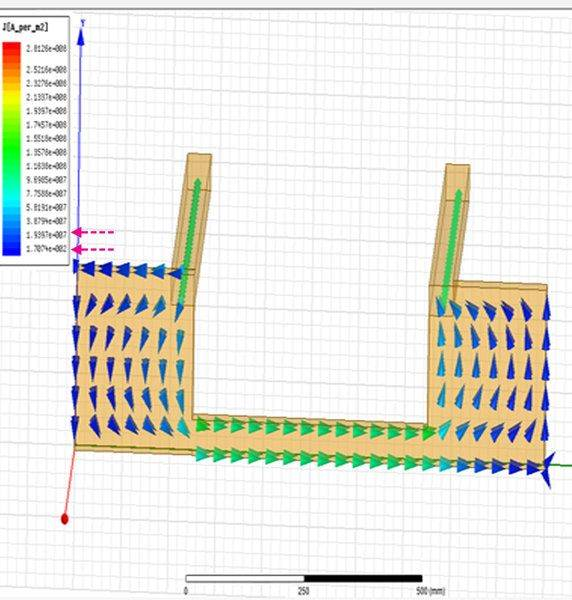# ANSYS - Maxwell, current distribution plot?

PhiowPhi
I was modeling current distribution in a component:And I plotted the ##\vec J## to simulate & understand the current distribution throughout the conductor, all the flows make sense, however, at the edge of the conductor like so:Current would distribute all around the area, which is expect. However, one would initially assume that the highest ##\vec J## would be going towards the potential difference applied(which is the lower right bar extending to the right of the page) which I would assume is ##1.93 \times 10^7##, and over the furthest left edges possibly low distribution at ##1.71 \times 10^2##, but I'm not sure. Is there a way I could figure this out through ANSYS?

Even when I apply really higher currents(From 250##A## to 250##kA##) the diagram would change to this distribution:Which leads to the same problem, dark blue being a range of [##1.73 \times 10^2##,##1.93 \times 10^7##] My initial assumption is the least amount of current in that scale list is towards the edges in dark-blue.